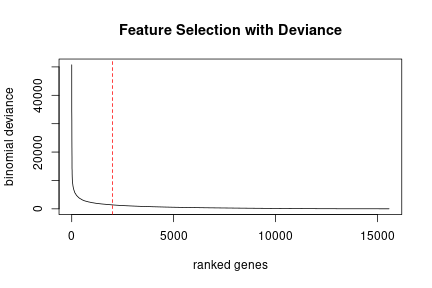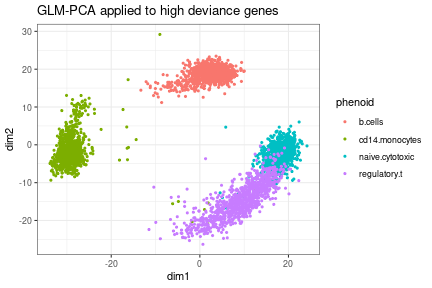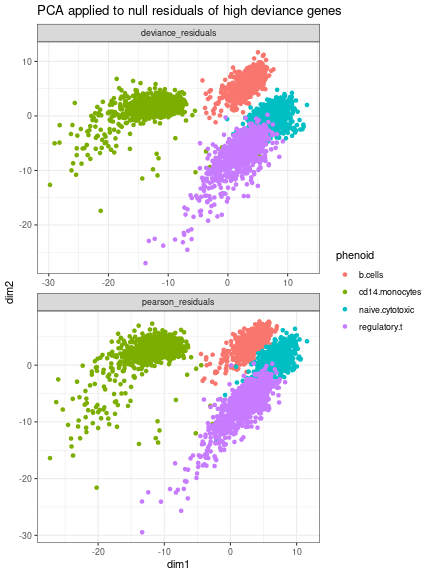# Basic Workflow

``````suppressPackageStartupMessages(library(SingleCellExperiment))
library(ggplot2); theme_set(theme_bw())
library(DuoClustering2018)
``````
``````## snapshotDate(): 2021-05-05
``````
``````require(scry)
``````

We illustrate the basic functionality of scry using a synthetic mixture of four known cell types from the DuoClustering2018 package.

``````sce<-sce_full_Zhengmix4eq()
``````
``````## see ?DuoClustering2018 and browseVignettes('DuoClustering2018') for documentation
``````
``````## loading from cache
``````
``````#m<-counts(sce) #UMI counts
#cm<-as.data.frame(colData(sce))
``````

## Feature Selection with Deviance

First we will rank genes based on deviance, to help identify the most biologically informative genes. The actual deviance values are stored in the rowData of the SingleCellExperiment object.

``````sce<-devianceFeatureSelection(sce, assay="counts", sorted=TRUE)
plot(rowData(sce)\$binomial_deviance, type="l", xlab="ranked genes",
ylab="binomial deviance", main="Feature Selection with Deviance")
abline(v=2000, lty=2, col="red")
``````We can see that the deviance drops sharply after about 2,000 genes. The remaining genes are probably not informative so we discard them to speed up downstream analysis.

``````sce2<-sce[1:1000, ]
``````

## Dimension Reduction with GLM-PCA

GLM-PCA can reduce the dimensionality of UMI counts to facilitate visualization and/or clustering without needing any normalization.

``````set.seed(101)
sce2<-GLMPCA(sce2, 2, assay="counts")
pd<-cbind(as.data.frame(colData(sce2)), fit\$factors)
ggplot(pd, aes(x=dim1, y=dim2, colour=phenoid)) + geom_point(size=.8) +
ggtitle("GLM-PCA applied to high deviance genes")
``````The separation between B-cells, monocytes, and T-cells is clear. The separation between naive cytotoxic and regulatory T-cells is less clear. Increasing the number of latent factors from 2 to 10 can improve resolution of biological clusters, but at the cost of slower computation.

## Dimension Reduction with Null Residuals

GLM-PCA can be slow for large datasets. A fast approximation is to fit a null model of constant expression for each gene across cells, then fit standard PCA to either the Pearson or deviance residuals from the null model.

``````sce<-nullResiduals(sce, assay="counts", type="deviance")
sce<-nullResiduals(sce, assay="counts", type="pearson")
sce2<-sce[1:1000, ] #use only the high deviance genes
pca<-function(Y, L=2, center=TRUE, scale=TRUE){
#assumes features=rows, observations=cols
res<-prcomp(as.matrix(t(Y)), center=center, scale.=scale, rank.=L)
factors<-as.data.frame(res\$x)
colnames(factors)<-paste0("dim", 1:L)
factors
}
pca_d<-pca(assay(sce2, "binomial_deviance_residuals"))
pca_d\$resid_type<-"deviance_residuals"
pca_p<-pca(assay(sce2, "binomial_pearson_residuals"))
pca_p\$resid_type<-"pearson_residuals"
cm<-as.data.frame(colData(sce2))
pd<-rbind(cbind(cm, pca_d), cbind(cm, pca_p))
ggplot(pd, aes(x=dim1, y=dim2, colour=phenoid)) + geom_point() +
facet_wrap(~resid_type, scales="free", nrow=2) +
ggtitle("PCA applied to null residuals of high deviance genes")
``````The null residuals approach still captures most of the biological structure, but the resolution between clusters is diminished and there is more noise.